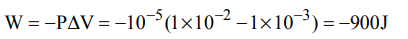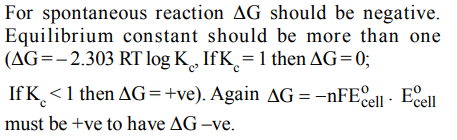## Thermodynamics Questions and Answers Part-10

1. The correct relationship between free energy change in a reaction and the corresponding equilibrium constant $K_{C}$ is
a) $-\triangle G =RT ln K_{C}$
b) $\triangle G^{\circ} =RT ln K_{C}$
c) $-\triangle G^{\circ} =RT ln K_{C}$
d) $\triangle G =RT ln K_{C}$

Explanation: $\triangle G^{\circ} = - RT ln K_{C}$    or $-\triangle G^{\circ} = RT ln K_{C}$

2. The enthalpy change for a reaction does not depend upon
a) use of different reactants for the same product
b) the nature of intermediate reaction steps
c) the differences in initial or final temperatures of involved substances
d) the physical states of reactants and products

Explanation: Enthalpy change for a reaction does not depend upon the nature of intermediate reaction steps.

3. An ideal gas expands in volume from $1×10^{-3}$  to $1×10^{-2}m^{3}$  at 300 K against a constant pressure of $1×10^{5}Nm^{-2}$  . The work done is
a) 270 kJ
b) – 300 kJ
c) – 900 kJ
d) 900 kJ

Explanation:4. The enthalpies of combustion of carbon and carbon monoxide are – 393.5 and – 283 kJ mol-1 respectively. The enthalpy of formation of carbon monoxide per mole is
a) – 676.5 kJ
b) 676.5 kJ
c) 110.5 kJ
d) – 110.5 kJ

Explanation:5. For a spontaneous reaction the $\triangle G$ , equilibrium constant (K) and $E\circ_{Cell}$ will be respectively
a) –ve, >1, –ve
b) –ve, <1, –ve
c) +ve, >1, –ve
d) –ve, >1, +ve

Explanation:6. Consider the reaction : $N_{2}+3H_{2}\rightarrow 2NH_{3}$    carried out at constant temperature and pressure. If $\triangle H and \triangle U$   are the enthalpy and internal energy changes for the reaction, which of the following expressions is true ?
a) $\triangle H > \triangle U$
b) $\triangle H < \triangle U$
c) $\triangle H = \triangle U$
d) $\triangle H =0$

Explanation:7. ($\triangle$H – $\triangle$U) for the formation of carbon monoxide (CO) from its elements at 298 K is (R = 8.314 J K–1 mol–1)
a) –2477.57 J mol–1
b) 2477.57 J mol–1
c) –1238.78 J mol–1
d) 1238.78 J mol–1

Explanation:8. An ideal gas is allowed to expand both reversibly and irreversibly in an isolated system. If $T_{i}$ is the initial temperature and $T_{f}$ is the final temperature, which of the following statements is correct?
a) $\left(T_{f}\right)_{rev}=\left(T_{f}\right)_{irrev}$
b) $\left(T_{f}\right)=\left(T_{i}\right)$   for both reversible and irreversible processes
c) $\left(T_{f}\right)_{irrev}>\left(T_{f}\right)_{rev}$
d) $\left(T_{f}\right)>\left(T_{i}\right)$   for reversible process but$T_{f}=T_{i}$   for irreversible process

Explanation: In a reversible process the work done is greater than in irreversible process. Hence the heat absorbed in reversible process would be greater than in the latter case. So Tf(rev.) < Tf(irr.)

9. The standard enthalpy of formation $\left(\triangle_{f}H^{\circ}\right)$ at 298 K for methane, $CH_{4}\left(g\right) is –74.8 kJ mol^{-1}$    . The additional information required to determine the average energy for C – H bond formation would be
a) the first four ionization energies of carbon and electron gain enthalpy of hydrogen
b) the dissociation energy of hydrogen molecule,$H_{2}$
c) the dissociation energy of $H_{2}$ and enthalpy of sublimation of carbon
d) latent heat of vapourization of methane

Explanation: The standard enthalpy of formation of CH4 is given by the equation : C(s) + 2H2 (g) $\rightarrow$ CH4 (g)
Hence, dissociation energy of hydrogen and enthalpy of sublimation of carbon is required.

10. The enthalpy changes for the following processes are listed below :
$Cl_{2}\left(g\right)=2Cl\left(g\right),242.3 kJ mol^{-1}$
$I_{2}\left(g\right)=2I\left(g\right),151.0 kJ mol^{-1}$
$ICl\left(g\right)=I\left(g\right)+Cl\left(g\right),211.3 kJ mol^{-1}$
$I_{2}\left(s\right)=I_{2}\left(g\right),62.76 kJ mol^{-1}$
Given that the standard states for iodine and chlorine are $I_{2}\left(s\right)andCl_{2}\left(g\right)$   , the standard enthalpy of formation for $ICl\left(g\right)$ is :
a) +16.8 kJ $mol^{-1}$
b) +244.8 kJ $mol^{-1}$
c) –14.6 kJ $mol^{-1}$
d) –16.8kJ $mol^{-1}$